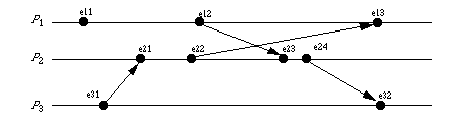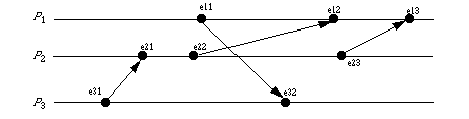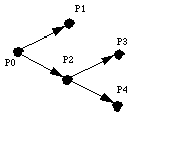# Distributed Systems Fundamentals

1. Distributed system?
1. What is it?
2. Why use it?
2. System Architectures
1. minicomputer model
2. workstation model
3. processor pool
3. Issues
1. global knowledge
2. naming
3. scalability
4. compatibility
5. process synchronization, communication
6. security
7. structure
4. Networks
1. goals
2. message, packet, subnet, session
3. switching: circuit, store-and-forward, message, packet, virtual circuit, dynamic routing
4. OSI model: PDUs, layering
1. physical: ethernet, aloha, etc.
3. network layer: virtual circult vs. datagram, routing via flooding, static routes, dynamic routes, centralized routing vs. distributed routing; congestion solutions (packet discarding, isarithmic, choke packets)
4. transport: services provided (UDP vs. TCP), functions to higher layers, addressing schemes (flat, DNS, etc.), gateway fragmentation and reassembly
5. session: adds session characteristics like authentication
6. presentation: compression, end-to-end encryption, virtual terminal
7. application: user-level programs
5. Clocks
1. happened-before relation
2. Lamport's distributed clocks: a -> b means C(a) < C(b)
3. Example where C(a) < C(b) does not mean a -> b
4. Vector clocks and causal relation
5. ordering of messages so you receive them in the order sent
1. why
3. for point to point: Schiper-Eggli-Sandoz
6. Global state
1. Show problem of slicing state when something is in transit
2. Define local state; send(mij) IN LSi iff time of send(mij) < current time of LSi; similar for receive
3. transit(LSi, LSj); inconsistent(LSi, LSj); consistent state is one with inconsistent set empty for all pairs LSi, LSj
4. Consistent global state: Chandry-Lamport
7. Termination detection

1. Haung

# Lamport's Clocks

## Introduction

Lamport's clocks keep a virtual time among distributed systems. The goal is to provide an ordering upon events within the system.

## Notation

• Pi process
• Ci clock associated with process Pi

## Protocol

1. Increment clock Ci between any two successive events in process Pi:
Ci <- Ci + d (d > 0)
2. Let event a be the sending of a message by process Pi; it is given the timestamp ta = C(a). Let b be the receipt of that message by Pj. Then when Pj receives the message,
Cj <- max(Cj, ta) + d (d > 0)

## ExampleAssume all clocks start at 0, and d is 1 (that is, each event incrememts the clock by 1). At event e12, C1(e12) = 2. Event e12 is the sending of a message to P2. When P2 receives the message (event e23), its clock C2 = 2. The clock is reset to 3. Event e24 is P2's sending a message to P3. That message is received at e32. C3 is 1 (as one event has passed). By rule 2, C3 is reset to the maximum of C2(e24)+1 and the current value of C3, so C3 becomes 5.

## Problem

Clearly, if a -> b, then C(a) < C(b). But if C(a) < C(b), does a -> b?

The answer, surprisingly, is not necessarily. In the above example, C3(e31) = 1 < 2 = C1(e12). But e31 and e12 are causally unrelated; that is, e31 ->X e12. However, C1(e11) < C3(e32), and clearly e11 -> e32. Hence one cannot say one way or the other.

# Vector Clocks

## Introduction

This is based upon Lamport's clocks, but each process keeps track of what is believes the other processes' interrnal clocks are (hence the name, vector clocks). The goal is to provide an ordering upon events within the system.

## Notation

• n processes
• Pi process
• Ci vector clock associated with process Pi; jth element is Ci[j] and contains Pi's latest value for the current time in process Pj

## Protocol

1. Increment clock Ci between any two successive events in process Pi:
Ci[i] <- Ci[i] + d (d > 0)
2. Let event a be the sending of a message by process Pi; it is given the vector timestamp ta = Ci(a). Let b be the receipt of that message by Pj. Then when Pj receives the message, it updates its vector clock for all k = 1, ..., n:
Cj[k] <- max(Cj[k], ta[k] + d) (d > 0)

## ExampleHere is the progression of time for the three processes:

e11: C1 = (1, 0, 0)
e31: C3 = (0, 0, 1)
e21: C2 = (0, 0, 1) as ta = C3(e31) = (0, 0, 1) and previously, C3 was (0, 0, 1)
e22: C2 = (0, 1, 1)
e12: C1 = (2, 0, 0)
e23: C2 = (2, 1, 1) as ta = C1(e12) = (2, 0, 0) and previously, C2 was (0, 1, 1)
e24: C2 = (2, 2, 1)
e13: C1 = (2, 1, 1) as ta = C2(e22) = (0, 1, 1) and previously, C1 was (2, 0, 0)
e32: C3 = (2, 2, 1) as ta = C2(e24) = (2, 2, 1) and previously, C3 was (0, 0, 1)

Notice that C1(e11) < C3(e32), so e11 -> e32, but C1(e11) and C3(e31) are incomparable, so e11 and e31 are concurrent.

# Birman-Schiper-Stephenson Protocol

## Introduction

The goal of this protocol is to preserve ordering in the sending of messages. For example, if send(m1) -> send(m2), then for all processes that receive both m1 and m2, receive(m1) -> receive(m2). The basic idea is that m2 is not given to the process until m1 is given. This means a buffer is needed for pending deliveries. Also, each message has an associated vector that contains information for the recipient to determine if another message preceded it. Also, we shall assume all messages are broadcast. Clocks are updated only when messages are sent.

## Notation

• n processes
• Pi process
• Ci vector clock associated with process Pi; jth element is Ci[j] and contains Pi's latest value for the current time in process Pj
• tm vector timestamp for message m (stamped after local clock is incremented)

## Protocol

Pi sends a message to Pj

1. Pi increments Ci[i] and sets the timestamp tm = Ci[i] for message m.

Pj receives a message from Pi

1. When Pj, j != i, receives m with timestamp tm, it delays the message's delivery until both:
1. Cj[i] = tm[i] - 1; and
2. for all k <= n and k != i, Cj[k] <= tm[k].
2. When the message is delivered to Pj, update Pj's vector clock
3. Check buffered messages to see if any can be delivered.

## ExampleHere is the protocol applied to the above situation:

e31: P3 sends message a; C3 = (0, 0, 1); ta = (0, 0, 1)
e21: P2 receives message a. As C2 = (0, 0, 0), C2 = ta - 1 = 1 - 1 = 0 and C2 => ta and C2 => ta = 0. So the message is accepted, and C2 is set to (0, 0, 1)
e11: P1 receives message a. As C1 = (0, 0, 0), C1 = ta - 1 = 1 - 1 = 0 and C1 => ta and C1 => ta = 0. So the message is accepted, and C1 is set to (0, 0, 1)
e22: P2 sends message b; C2 = (0, 1, 1); tb = (0, 1, 1)
e12: P1 receives message b. As C1 = (0, 0, 1), C1 = tb - 1 = 1- 1 = 0 and C1 => tb and C1 => tb = 0. So the message is accepted, and C1 is set to (0, 1, 1)
e32: P3 receives message b. As C3 = (0, 0, 1), C3 = tb - 1 = 1 - 1 = 1 and C1 => tb and C1 => tb = 0. So the message is accepted, and C3 is set to (0, 1, 1)

Now, suppose ta arrived as event e12, and tb as event e11. Then the progression of time in P1 goes like this:

e11: P1 receives message b. As C1 = (0, 0, 0), C1 = tb - 1 = 1 - 1 = 0 and C1 => tb, but C1 < tb, so the message is held until another message arrives. The vector clock updating algorithm is not run.
e12: P1 receives message a. As C1 = (0, 0, 0), C1 = ta - 1 = 1 - 1 = 0, C1 => ta, and C1 => ta. The message is accepted and C1 is set to (0, 0, 1). Now the queue is checked. As C1 = tb - 1 = 1 - 1 = 0, C1 => tb, and C1 => tb, that message is accepted and C1 is set to (0, 1, 1).

# Schiper-Eggli-Sandoz Protocol

## Introduction

The goal of this protocol is to ensure that messages are given to the receiving processes in order of sending. Unlike the Birman-Schiper-Stephenson protocol, it does not require using broadcast messages. Each message has an associated vector that contains information for the recipient to determine if another message preceded it. Clocks are updated only when messages are sent.

## Notation

• n processes
• Pi process
• Ci vector clock associated with process Pi; jth element is Ci[j] and contains Pi's latest value for the current time in process Pk
• tm vector timestamp for message m (stamped after local clock is incremented)
• ti current time at process Pi
• Vi vector of Pi's previously sent messages; Vi[j] = tm, where Pj is the destination process and tm the vector timestamp of the message; Vi[j][k] is the kth component of Vi[j].
• Vm vector accompanying message m

## Protocol

Pi sends a message to Pj

1. Pi sends message m, timestamped tm, and Vi, to process Pj
2. Pi sets Vi[j] = tm

Pj receives a message from Pi

1. When Pj, j != i, receives m, it delays the message's delivery if both:
1. Vm[j] is set; and
2. Vm[j] < tj
2. When the message is delivered to Pj, update all set elements of Vj with the corresponding elements of Vm, except for Vj[j], as follows:
1. If Vj[k] and Vm[k] are uninitialized, do nothing.
2. If Vj[k] is uninitialized and Vm[k] is initialized, set Vj[k] = Vm[k].
3. If both Vj[k] and Vm[k] are initialized, set Vj[k][k'] = max(Vj[k][k'], Vm[k][k']) for all k' = 1, ..., n
3. Update Pj's vector clock.
4. Check buffered messages to see if any can be delivered.

## ExampleHere is the protocol applied to the above situation:

e31: P3 sends message a to P2. C3 = (0, 0, 1); ta = (0, 0, 1), Va = (?, ?, ?); V3 = (?, (0, 0, 1), ?)
e21: P2 receives message a from P1. As Va is uninitialized, the message is accepted. V2 is set to (?, ?, ?) and C2 is set to (0, 0, 1).
e22: P2 sends message b to P1. C2 = (0, 1, 1); tb = (0, 1, 1), Vb = (?, ?, ?); V2 = ((0, 1, 1), ?, ?)
e11: P1 sends message c to P3. C1 = (1, 0, 0); tc = (1, 0, 0), Vc = (?, ?, ?); V1 = (?, ?, (1, 0, 0))
e12: P1 receives message b from P2. As Vb is uninitialized, the message is accepted. V1 is set to (?, ?, ?) and C1 is set to (1, 1, 1).
e32: P3 receives message c from P1. As Vc is uninitialized, the message is accepted. V3 is set to (?, ?, ?) and C3 is set to (1, 0, 1).
e23: P2 sends message d to P1. C2 = (0, 2, 1); td = (0, 2, 1), Vd = ((0, 1, 1), ?, ?); V2 = ((0, 2, 1), ?, (0, 0, 1))
e13: P1 receives message d from P2. As Vd < C1, so the message is accepted. V1 is set to ((0, 1, 1), ?, ?) and C1 is set to (1, 2, 1).

Now, suppose tb arrived as event e13, and td as event e12. Then the progression in P1 goes like this:

e12: P1 receives message d from P2. But Vd = (0, 1, 1) <X (1, 0, 0) = C3, so the message is queued for later delivery.
e13: P1 receives message b from P2. As Vb is uninitialized, the message is accepted. V1 is set to (?, ?, ?) and C1 is set to (1, 1, 1). The message on the queue is now checked. As Vd = (0, 1, 1) < (1, 1, 1) = C1, the message is now accepted. V1 is set to ((0, 1, 1), ?, ?) and C1 is set to (1, 2, 1).

# Chandy-Lamport Global State Recording Protocol

## Introduction

The goal of this distributed algorithm is to capture a consistent global state. It assumes all communication channels are FIFO. It uses a distinguished message called a marker to start the algorithm.

## Protocol

Pi sends marker

1. Pi records its local state LSi
2. For each Cij on which Pi has not already sent a marker, Pi sends a marker before sending other messages.

1. If Pi has not recorded its state:
1. Record the state of Cji as empty
2. Send the marker as described above
2. If Pi has recorded its state LSi
1. Record the state of Cji to be the sequence of messages received between the computation of LSi and the marker from Cji.

## ExampleHere, all processes are connected by communications channels Cij. Messages being sent over the channels are represented by arrows between the processes.

Snapshot s1:

P1 records LS1, sends markers on C12 and C13
P2 receives marker from P1 on C12; it records its state LS2, records state of C12 as empty, and sends markers on C21 and C23.
P3 receives marker from P1 on C13; it records its state LS3, records state of C13 as empty, and sends markers on C31 and C32.
P1 receives marker from P2 on C21; as LS1 is recorded, it records the state of C21 as empty.
P1 receives marker from P3 on C31; as LS1 is recorded, it records the state of C31 as empty.
P2 receives marker from P3 on C32; as LS2 is recorded, it records the state of C32 as empty.
P3 receives marker from P2 on C23; as LS3 is recorded, it records the state of C23 as empty.

Snapshot s2: now a message is in transit on C12 and C21.

P1 records LS1, sends markers on C12 and C13
P2 receives marker from P1 on C12 after the message from P1 arrives; it records its state LS2, records state of C12 as empty, and sends marker on C21 and C23
P3 receives marker from P1 on C13; it records its state LS3, records state of C13 as empty, and sends markers on C31 and C32.
P1 receives marker from P2 on C21; as LS1 is recorded, and a message has arrived since LS1 was recorded, it records the state of C21 as containing that message.
P1 receives marker from P3 on C31; as LS1 is recorded, it records the state of C31 as empty.
P2 receives marker from P3 on C32; as LS2 is recorded, it records the state of C32 as empty.
P3 receives marker from P2 on C23; as LS3 is recorded, it records the state of C23 as empty.

# Huang's Termination Detection Protocol

## Introduction

The goal of this protocol is to detect when a distributed computation terminates.

## Notation

• n processes
• Pi process; without loss of generality, let P0 be the controlling agent
• Wi weight of process Pi; initially, W0 = 1 and for all other i, Wi = 0.
• B(W) computation message with assigned weight W
• C(W) control message sent from process to controlling agent with assigned weight W

## Protocol

Pi sends a computation message to Pj

1. Set Wi' and Wj to values such that Wi' + Wj = Wi, Wi > 0, Wj > 0. (Wi' is the new weight of Pi.)
2. Send B(Wj) to Pj

Pj receives a computation message B(W) from Pi

1. Wj <- Wj + W
2. If Pj is idle, Pj becomes active

Pi becomes idle

1. Send C(Wi) to P0
2. Wi = 0
3. Pi becomes idle

Pi receives a control message C(W)

1. Wi <- Wi + W
2. If Wi = 1, the computation has completed.

## Example

The picture shows a process P0, designated the controlling agent, with W0 = 1. It asks P1 and P2 to do some computation.It sets W1 to 0.2, W2 to 0.3, and W3 to 0.5. P2 in turn asks P3 and P4 to do some computations. It sets W3 to 0.1 and W4 to 0.1.

When P3 terminates, it sends C(W3) = C(0.1) to P2, which changes W2 to 0.1 + 0.1 = 0.2.

When P2 terminates, it sends C(W2) = C(0.2) to P0, which changes W0 to 0.5 + 0.2 = 0.7.

When P4 terminates, it sends C(W4) = C(0.1) to P0, which changes W0 to 0.7 + 0.1 = 0.8.

When P1 terminates, it sends C(W1) = C(0.2) to P0, which changes W0 to 0.8 + 0.2 = 1.

P0 thereupon concludes that the computation is finished.

Total number of messages passed: 8 (one to start each computation, one to return the weight).Send email to cs251@csif.cs.ucdavis.edu.

Department of Computer Science
University of California at Davis
Davis, CA 95616-8562